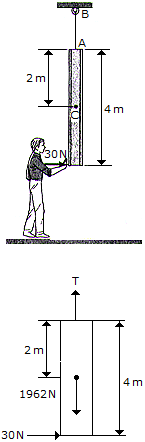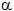# Engineering Mechanics - PKRB: Force and Animation - Discussion

### Discussion :: PKRB: Force and Animation - General Questions (Q.No.1)

1.The slender 200-kg beam is suspended by a cable at its end as shown. If a man pushes on its other end with a horizontal force of 30 N, determine the initial acceleration of its mass center G, the beam's angular acceleration, and the tension in the cable AB.

 [A]. aG = 0,= 0.225 rad/s2, T = 1.962 kN [B]. aG = 0.0750 m/s2,= 0.1125 rad/s2, T = 1.962 kN [C]. aG = 0,= 0.1125 rad/s2, T = 1.962 kN [D]. aG = 0.1500 m/s2,= 0.225 rad/s2, T = 1.962 kN

Explanation:

No answer description available for this question.

 Amadu Kwabena said: (Jan 12, 2019) How? Explain the answer in detail.